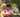### Max Product Of Three

January 27, 2020

Max Product Of Three

A non-empty array A consisting of N integers is given. The product of triplet (P, Q, R) equates to A[P] * A[Q] * A[R] (0 ≤ P < Q < R < N).

For example, array A such that:
A = -3
A = 1
A = 2
A = -2
A = 5
A = 6
contains the following example triplets:
• (0, 1, 2), product is −3 * 1 * 2 = −6
• (1, 2, 4), product is 1 * 2 * 5 = 10
• (2, 4, 5), product is 2 * 5 * 6 = 60
Your goal is to find the maximal product of any triplet.

Write a function:
function solution(A);
that, given a non-empty array A, returns the value of the maximal product of any triplet.
For example, given array A such that:
A = -3
A = 1
A = 2
A = -2
A = 5
A = 6
the function should return 60, as the product of triplet (2, 4, 5) is maximal.

Write an efficient algorithm for the following assumptions:
• N is an integer within the range [3..100,000];
• each element of array A is an integer within the range [−1,000..1,000].

``````function solution(A) {
// write your code in JavaScript (Node.js 8.9.4)
A.sort((a,b)=>a-b)
//console.log("sorted: ",A)

const L = A.length

//possible max products:
//1.first two negative numbers * the last positive number
//or 2.last three positive numbers
const max = Math.max(A*A*A[L-1], A[L-3]*A[L-2]*A[L-1])

return max
}``````Posted by Tai Lu ( 呂台生 ) who's building useful things.You should follow him on Twitter